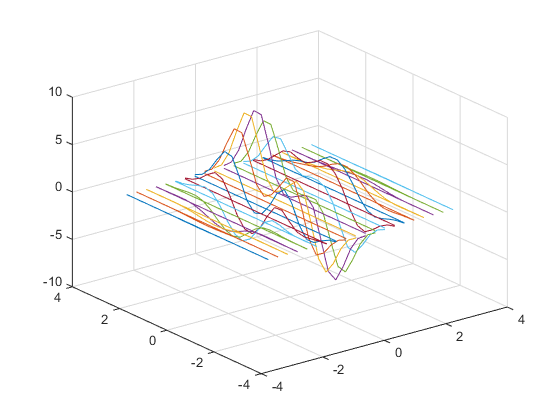Documentation

## Responding to Hold State

This example shows how to test for `hold` state and respond appropriately in user-defined plotting functions.

Plotting functions usually change various axes parameters to accommodate different data. The `myPlot3D` function:

• Uses a 2-D or 3-D view depending on the input data.

• Respects the current `hold` state, to be consistent with the behavior of MATLAB® plotting functions.

```function myPlot3D(x,y,z) % Call newplot to get the axes handle cax = newplot; % Save current hold state hold_state = ishold; % Call plotting commands to % produce custom graph if nargin == 2 line(x,y); % Change view only if hold is off if ~hold_state view(cax,2) end elseif nargin == 3 line(x,y,z); % Change view only if hold is off if ~hold_state view(cax,3) end end grid on end```

For example, the first call to `myPlot3D` creates a 3-D graph. The second call to `myPlot3D` adds the 2-D data to the 3-D view because `hold` is `on`.

```[x,y,z] = peaks(20); myPlot3D(x,y,z) hold on myPlot3D(x,y)```